## What is the delta H when 72.0 grams H2O condenses at 100.00C? Here are some constants that you MAY need. specific

Question

What is the delta H when 72.0 grams H2O condenses at 100.00C?

Here are some constants that you MAY need.

specific heats heat of fusion heat of vaporization

H2O(s) = 2.1 J/g0C 6.01 kJ/mole 40.7 kJ/mole

H2O(L) = 4.18 J/g0C

H2O(g) = 1.7 J/g0C

2930 kJ

163 kJ

-163 kJ

-2930 kJ

in progress 0
2 months 2021-07-22T15:48:17+00:00 1 Answers 7 views 0

1. Answer: The value of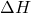is -163 kJZ

Explanation:

The number of moles is defined as the ratio of the mass of a substance to its molar mass. The equation used is: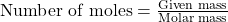……(1)

We are given:

Given mass of water = 72.0 g

Molar mass of water = 18 g/mol

Putting values in equation 1, we get: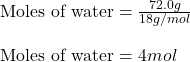Calculating the heat released for the condensation process: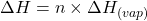……(2)

where,= amount of heat released

n = number of moles of water = 4 moles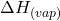= specific heat of vaporization = -40.7 kJ/mol

Negative sign represents the amount of heat released.

Putting values in equation 2: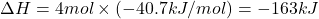Hence, the value ofis -163 kJ